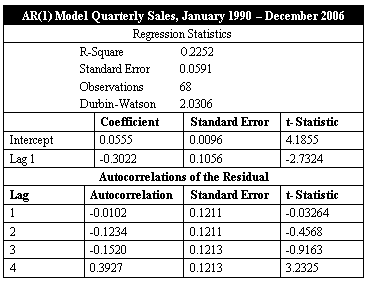### CFA Practice Question

There are 208 practice questions for this study session.

### CFA Practice Question

The following table gives the regression output of an AR(1) model.Which statement is true about the time series?

I. There is a strong seasonal autocorrelation of the residuals.
II. The quarterly sales amount looks like a random walk.
III. We cannot reject the null hypothesis that the fourth autocorrelation is equal to 0.
IV. The intercept differs significantly from 0.
A. I and III
B. II and IV
C. I and IV
Explanation: The critical value for a t-statistic is about 2.0 at the 0.05 significance level and 66 degrees of freedom. The lag 1, intercept and fourth autocorrelation all have a t-statistics that are larger than 2.0 so we can reject the null hypothesis that they are 0.